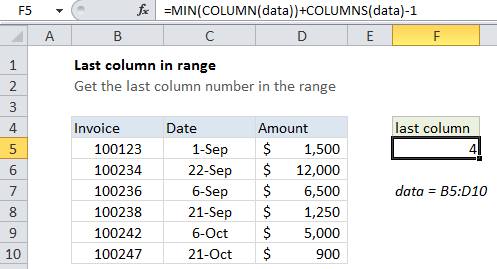## Excel Office

Excel How Tos, Tutorials, Tips & Tricks, Shortcuts

# How to get last column number in range in Excel

You can get the last column in a range with a formula based on the COLUMN function. See example below:

## Formula

`=MIN(COLUMN(range))+COLUMNS(range)-1`Note: When given a single cell reference, the COLUMN function returns the column number for that reference. However, when given a range that contains multiple columns, the COLUMN function will return an array that contains all column numbers for the range.

## Explanation

In the example shown, the formula in cell F5 is:

`=MIN(COLUMN(data))+COLUMNS(data)-1`

where data is a named range for B5:D10

Worked Example:   Minimum value if in Excel

### How this formula works

If you want only the first column number, you can use the MIN function to extract just the first column number, which will be the lowest number in the array.

Once we have the first column, we can just add the total columns in the range and subtract 1, to get the last column number.

Worked Example:   How to get address of named range in Excel

### Index version

Instead of MIN, you can also use INDEX to get the last row number:

`=COLUMN(INDEX(data,1,1))+COLUMNS(data)-1`

This is possibly a bit faster for large ranges, since INDEX just supplied a single cell to COLUMN.

### Simple version

When a formula returns an array result, Excel will display the first item in the array if the formula is entered in a single cell. This means that in practice, you can often just use a simplified version of the formula:

`=COLUMN(data)+COLUMNS(data)-1`

But be aware that this will return an array for a multi-column range.

Worked Example:   How to get relative row numbers in a range in Excel

Inside formulas, it’s sometimes necessary to make sure you are dealing with only one item, and not an array. In that case, you’ll want to use the full version above.